Author: Oscar Cronquist Article last updated on August 19, 2021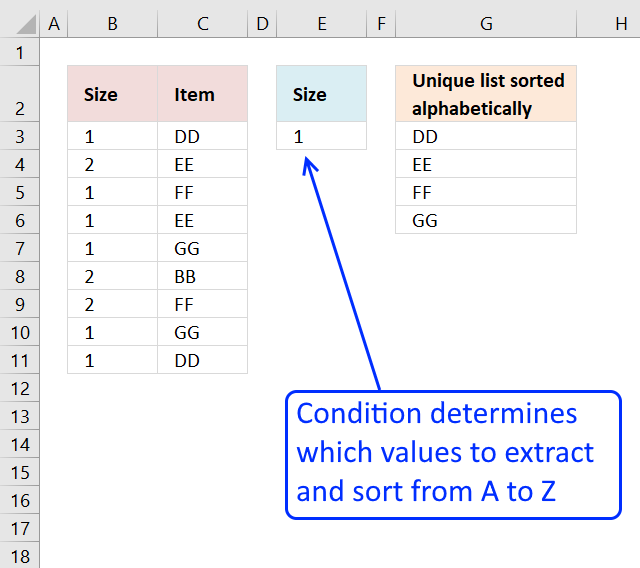This article demonstrates how to extract unique distinct values based on a condition and also sorted from A to z.

## 1. Unique distinct list sorted alphabetically based on a condition - earlier Excel versions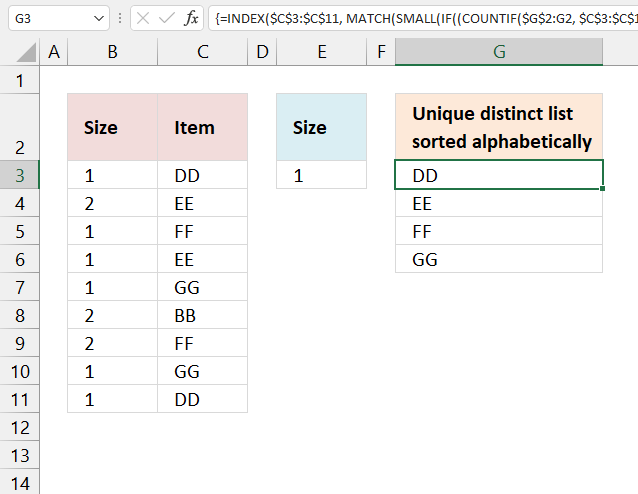The image above demonstrates a formula in cell G6 that extracts unique distinct values only if the corresponding value on the same row meets a given condition.

The array formula below is for earlier Excel versions, it filters values in column C based on the value in cell E3, the output is a sorted unique distinct list in cell G3 and cells below.

Array formula in cell G3:

=INDEX(\$C\$3:\$C\$11, MATCH(SMALL(IF((COUNTIF(\$G\$2:G2, \$C\$3:\$C\$11)=0)*(\$B\$3:\$B\$11=\$E\$3), COUNTIF(\$C\$3:\$C\$11, "<"&\$C\$3:\$C\$11), ""), 1), COUNTIF(\$C\$3:\$C\$11, "<"&\$C\$3:\$C\$11), 0))

Recommended post

Recommended articles

### 1.1 How to create an array formula

1. Double press with left mouse button on cell G3.
2. Copy (Ctrl + c) and paste (Ctrl + v) array formula to cell.
3. Press and hold CTRL + SHIFT simultaneously.
4. Press Enter once.
5. Release all keys.

There is now a beginning and ending curly bracket in the formula bar, like this: {=formula}
Don't enter these characters yourself, they appear automatically if you completed the above steps.

### 1.2 Explaining array formula in cell G3

#### Step 1 - Count values

The COUNTIF function counts values based on a condition or criteria.

COUNTIF(rangecriteria)

The range argument contains a cell reference that is both relative and absolute meaning it grows when the formula is copied to cells below. This makes the formula aware of the previous values above.

COUNTIF(\$G\$2:G2, \$C\$3:\$C\$11)

becomes

COUNTIF("Unique distinct list sorted alphabetically",{"DD";"EE";"FF";"EE";"GG";"BB";"FF";"GG";"DD"})

and returns {0;0;0;0;0;0;0;0;0}. 0 (zero) means that no cells meet the given condition.

#### Step 2 - Check if values in array equals 0 (zero)

The equal sign compares the values in the array to 0 (zero). The output is a boolean value TRUE or FALSE.

COUNTIF(\$G\$2:G2, \$C\$3:\$C\$11)=0

becomes

{0;0;0;0;0;0;0;0;0}=0

and returns {TRUE; TRUE; TRUE; TRUE; TRUE; TRUE; TRUE; TRUE; TRUE}.

\$B\$3:\$B\$11=\$E\$3

#### Step 4 -

COUNTIF(\$G\$2:G2, \$C\$3:\$C\$11)=0)*(\$B\$3:\$B\$11=\$E\$3)

#### Step 5 -

IF((COUNTIF(\$G\$2:G2, \$C\$3:\$C\$11)=0)*(\$B\$3:\$B\$11=\$E\$3), COUNTIF(\$C\$3:\$C\$11, "<"&\$C\$3:\$C\$11), "")

#### Step 6 -

SMALL(IF((COUNTIF(\$G\$2:G2, \$C\$3:\$C\$11)=0)*(\$B\$3:\$B\$11=\$E\$3), COUNTIF(\$C\$3:\$C\$11, "<"&\$C\$3:\$C\$11), ""), 1)

#### Step 7 -

MATCH(SMALL(IF((COUNTIF(\$G\$2:G2, \$C\$3:\$C\$11)=0)*(\$B\$3:\$B\$11=\$E\$3), COUNTIF(\$C\$3:\$C\$11, "<"&\$C\$3:\$C\$11), ""), 1), COUNTIF(\$C\$3:\$C\$11, "<"&\$C\$3:\$C\$11), 0)

#### Step 8 -

INDEX(\$C\$3:\$C\$11, MATCH(SMALL(IF((COUNTIF(\$G\$2:G2, \$C\$3:\$C\$11)=0)*(\$B\$3:\$B\$11=\$E\$3), COUNTIF(\$C\$3:\$C\$11, "<"&\$C\$3:\$C\$11), ""), 1), COUNTIF(\$C\$3:\$C\$11, "<"&\$C\$3:\$C\$11), 0))

Read my explanation here: Create a unique distinct alphabetically sorted list, extracted from a column

Recommended articles

## 2. Unique distinct list sorted alphabetically based on a condition - Excel 365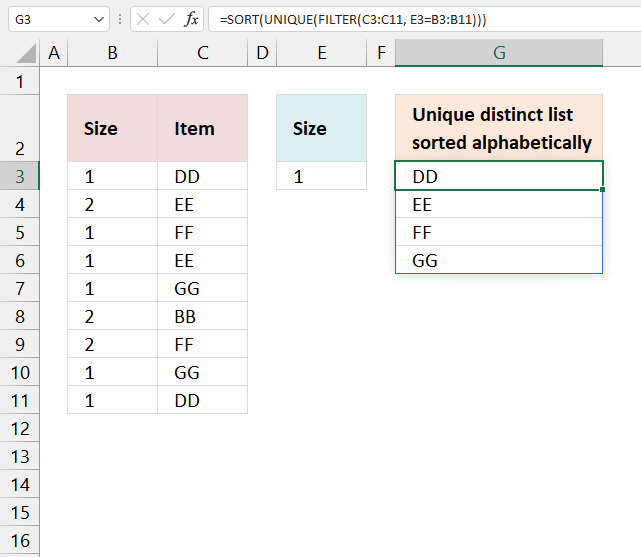Update 17 December 2020, the new FILTER, UNIQUE, and SORT functions are now available for Excel 365 users.

The image above demonstrates a dynamic array formula that works only in Excel 365. Despite its name you simply enter it as a regular formula.

It filters values from column C based on the corresponding values in column B given the condition in cell E3. The output in cell G3 is a sorted unique distinct list from A to Z.

=SORT(UNIQUE(FILTER(C3:C11, E3=B3:B11)))

This is entered as a regular formula, however, it returns an array of values and extends automatically to cells below and to the right. Microsoft calls this a dynamic array and spilled array.

### 2.1 Explaining Excel 365 formula

#### Step 1 - Extract values based on a condition

The FILTER function extracts values based on a condition.

FILTER(C3:C11, E3=B3:B11)

#### Step 2 - Extract unique distinct values

The UNIQUE function returns an array of unique distinct values meaning duplicates are merged into one distinct value.

UNIQUE(FILTER(C3:C11, E3=B3:B11))

#### Step 3 - Sort values from A to Z

The SORT function returns an array of values sorted from A to Z in its default state.

SORT(UNIQUE(FILTER(C3:C11, E3=B3:B11)))

## 3. Unique distinct list sorted alphabetically based on a range - Earlier Excel versions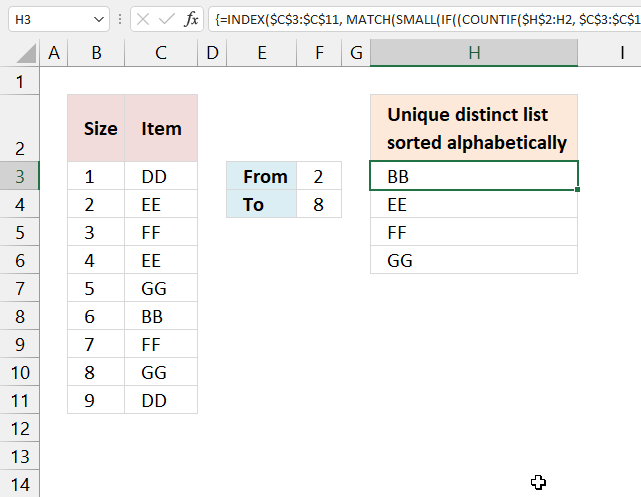The image above shows a formula in cell H3 that extracts values from column C it the corresponding numbers in column B meet a condition based on a numerical range specified in cells F3:F4.

The output in cell H3 and cells below as far as needed is a sorted unique distinct list from A to Z. Unique distinct values are all values, however, duplicate values are merged into one distinct value.

Formula in cell H3:

=INDEX(\$C\$3:\$C\$11, MATCH(SMALL(IF((COUNTIF(\$H\$2:H2, \$C\$3:\$C\$11)=0)*(\$B\$3:\$B\$11>=\$F\$3)*(\$B\$3:\$B\$11<=\$F\$4), COUNTIF(\$C\$3:\$C\$11, "<"&\$C\$3:\$C\$11), ""), 1), COUNTIF(\$C\$3:\$C\$11, "<"&\$C\$3:\$C\$11), 0))

## 4. Unique distinct list sorted alphabetically based on a range - Excel 365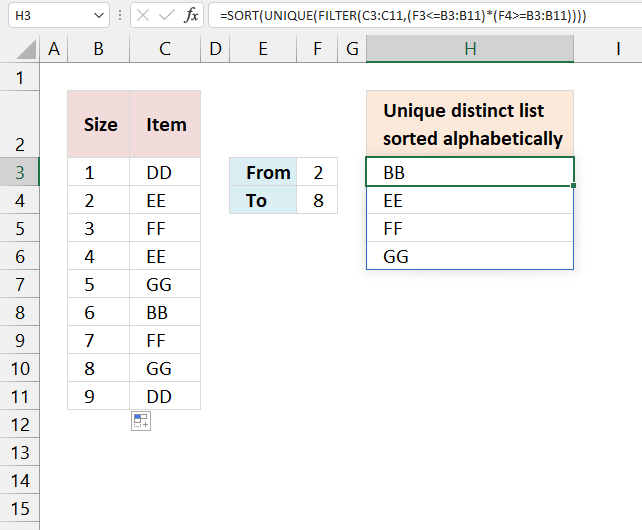Formula in cell H3:

=SORT(UNIQUE(FILTER(C3:C11,(F3<=B3:B11)*(F4>=B3:B11))))

### 4.1 Explaining Excel 365 formula

F3<=B3:B11

F4>=B3:B11

#### Step 3 -

(F3<=B3:B11)*(F4>=B3:B11)

#### Step 4 -

FILTER(C3:C11,(F3<=B3:B11)*(F4>=B3:B11))

#### Step 5 -

UNIQUE(FILTER(C3:C11,(F3<=B3:B11)*(F4>=B3:B11)))

#### Step 6 -

SORT(UNIQUE(FILTER(C3:C11,(F3<=B3:B11)*(F4>=B3:B11))))